ELECTRICAL PROPERTIES BASICS
Electrical properties are terms used in the Archie water saturation equation, and in its shale corrected cousins, to calibrate water saturation to the physical properties of the pore geometry of the rock. The parameters required are:
1: tortuosity constant A
2: cementation exponent M
3: saturation exponent N

These three terms are usually written in lower case font (a, m, n) but in keeping with the policy of this Handbook that input parameters be capitalized, they are referred to here and elsewhere in upper caseM is defined as the slope of the best fit line drawn through a graph of formation factor (F = R0 / RW) versus porosity,  and A is the intercept of that line on the F axis. A sample of such a plot is show at the left and is discussed in more detail later in this Chapter.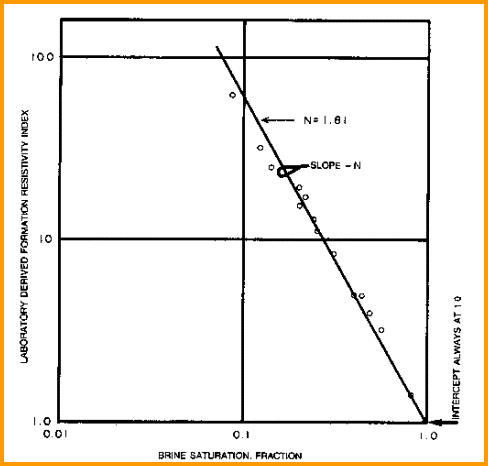N is defined as the slope of the best fit line on a graph of resistivity index (RI = RT / R0) versus water saturation. A sample plot is shown at the right and discussed in more detail later..

Although there is not a lot of evidence to support the assertion, N is often taken as equal to M, probably because M can sometimes be found from available log data in the absence of core data, and N cannot.

Pore geometry in sandstones varies with lithology, grain size, sorting, shape, roughness, and shale volume. In carbonates, it varies with pore type and how well the pores are connected. Some examples are shown below, and combinations of these pore geometries are common. For example, fractured, moldic, intercrystalline porosity is relatively common.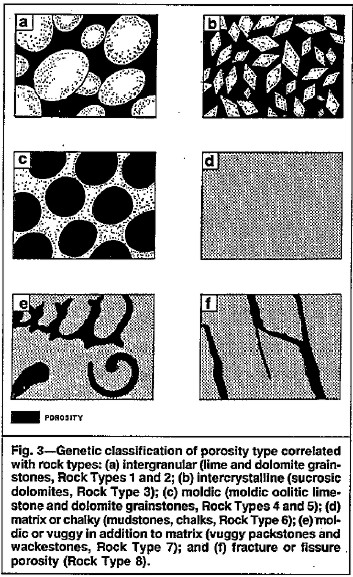Examples of different pore geometry models

The cementation exponent has been studied the most and the following illustrations, keyed to the pore geometry shown above, give some idea of the complexity of the problem. The two images below are from "Cementation Exponents in Middle Eastern Carbonate Reservoirs" by J. W. Focke and D. Munn, SPE Paper 13175, June 1987.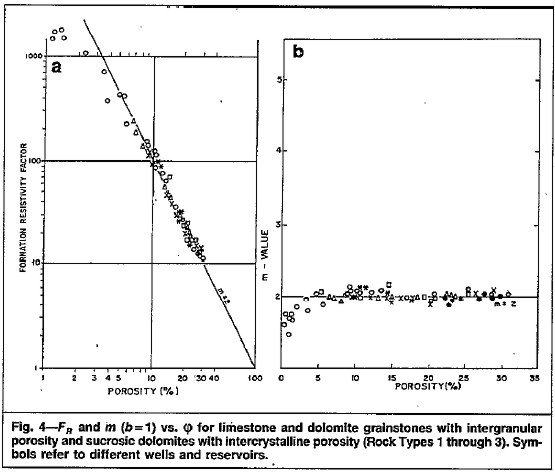Formation factor plot and M versus porosity plot for intergranular and sucrosic pore geometry.
A = 1.00, M = 2.00, and do not vary with porosity.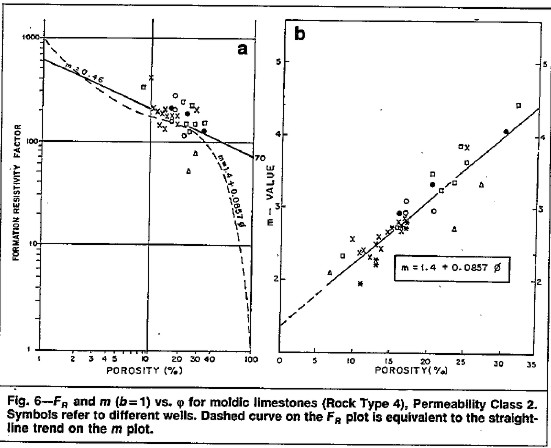Similar plots for a moldic limestone. Formation factor data is scattered and M increases with
increasing porosity. A variable M method is required for a satisfactory water saturation calculation.
The equation for M is: M = 1.40 + 6.57 * PHIe where PHIe is a decimal fraction.
(permeability
regime 0.1 - 1.0 md)

The slope and intercept of the variable M plots will of course vary from one reservoir to another, and vary with the permeability regime, which is an indicator of the connectedness of the moldic porosity.

There are a number of methods for finding and adjusting the electrical properties of rocks. The common approaches are listed here.Tortuosity Constant (A) from Log and RW Data
This is easy if there is a clean water zone on the log which also tested water. The formula is:     1: Rwa = (PHIt ^ M) * RESD / A
1: Rwa = (PHIt ^ 2) * RESD
2: RW@FTlog = Rwa from a relatively clean water bearing zone
3: KT1 = 6.8 for English units    KT1 = 21.5 for Metric units
4: RW@FTdst = RWlab * (TRW + KT1) / (FT + KT1)
5: A = (RW@FTlog) / (RW@FTdst)

Where:
A = cementation exponent (unitless)
RW@FTlog = RW@FT with A = 1.0 and M = 2.0 calculated from a water zone on logs (ohm-m)
RW@FTdst = RW@FT from DST measured in the laboratory (ohm-m) and converted to formation temperature
FT = formation temperature (degrees Fahrenheit or Celsius)
RWlab = water resistivity at lab temperature (ohm-m)
TRW = temperature at which water was measured (degrees Fahrenheit or Celsius)

Data from several wells may be averaged to obtain a good value. The parameter A varies with grain size, so wells used should come from similar geologic settings (depth, distance from source of sediment, etc.).Cementation Exponent (M) from Special Core Data
Plot porosity vs lab measured formation factor on log-log axes. Fit regression or eyeball line to data. Slope of line is M. Intercept at PHIe = 1 is A. The line force-fitted through F = PHIe = 1.0 is called a pinned line. Some people prefer the pinned line but most data sets do not support this approach. Strictly speaking, the line must pass through F = 1 = PHIe, so the line must be non-linear approaching this point on the graph. An example is shown below.Find A and M from special core data (electrical properties data) - M is slope of best fit line
(pinned or free regression - your choice), A is intercept at PHIe = 1.0.Saturation Exponent (N) from Special Core Data
Plot brine saturation from core analysis versus formation resistivity index (RESD / R0) on log-log axes. Draw line through the data to intercept at SW = 1.0. The slope of this line is N. Data from several wells may have to be combined to get a reasonable fit.Find N from special core data (electrical properties data). Slope is N and line must pass
through Sw = 1.0 at RI = 1.0..Sample Special Core REPORTS
The graphs described above are provided by the core analysis contractor based on the measurements they have made. These data are also provided as a table or spreadsheet. The layout may vary from the examples shown here. You are free to edit or combine data sets and make your own graphs and determine new A, M, and N values specific to a particular zone, facies, or rock type.The final product from the lab includes formation factor and resistivity graphs and  a table of F, M, RI, and N values for use in the appropriate water saturation equations. This example is from a clean, moderately tight, dolomitic sandstone.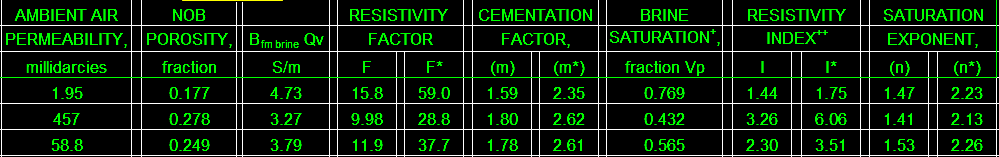A shaly sand special core analysis gives a table of BQv, F, F*, M, M*, RI, RI*, N, and N* for use in the appropriate water saturation equations.This example is from a Cretaceous shaly sand in southern Alberta.Cementation Exponent (M) from Log Data
A variety of approaches are available to assess the electrical property M from log data. There is no direct method for finding N from log data, but there is an indirect approach. If you are satisfied that A, M, and RW are correct, you can compare the log analysis SW with the capillary pressure SW. Any difference can be repaired by adjusting N. Changing N will make no difference in a water zone, so it helps to be able to calibrate A, M, and RW in the water zones first.Pickett Plot Method
Pickett proposed that the Archie formation factor and saturation equations be rearranged as follows:
2: log(RESD) = - M * log(PHIe) + log(A*RW@FT)
3: log(RESS) = - M * log(PHIe) + log(A*RMF@FT)

In water zones:
4: M = (log(A*RW@FT) - log(RESD)) / log(PHIe)

In invaded water or hydrocarbon zones:
5: M = (log(A*RMF@FT) - log(RESS)) / log(PHIe)

Where:
A = tortuosity exponent (unitless)
M = cementation exponent (unitless)
PHIe = porosity from any source (fractional
RESD = deep resistivity of rock with water and oil (or mercury) (ohm-m)
RESS = shallow resistivity of rock with water and oil (or mercury) (ohm-m)
RW@FT = water resistivity at formation temperature (ohm-m)
RMF@FT = mud filtrate resistivity at formation temperature (ohm-m)COMMENTS
Equation 1 represents a plot of deep resistivity vs effective porosity FROM KNOWN WATER ZONES on log-log paper. Draw a line through southwest data points. Slope of this line is M and intercept at PHIe = 1 is A*RW@FT.

Equation 2 may work in hydrocarbon zones if invasion is well developed and residual hydrocarbon is small. M will be too high if ROS is high.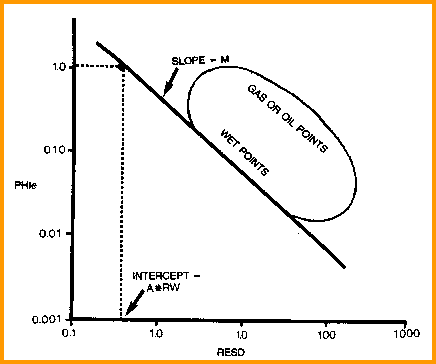Find M and A*RW from Pickett plot

Make a separate graph FOR EACH ROCK TYPE. Typical rock types in carbonates are intergranular (clastic texture), intercrystalline (fine, medium, or coarse), vuggy (fine, medium, or large), microporosity (unconnected pores), oomoldic, and fractured zones (with any other rock type). Rock typing is usually done from sample or core description.

M can be made to vary by solving equation 1 or 2 for each data point instead of fitting a line through the average of the data set.

/"VARIABLE M" METHODSShell Method
Analysts at Shell Oil proposed a formula to vary M in carbonates with porosity. Other relationships could be found by fitting non-linear curves to the data used for the Pickett plot or by plotting individual M values versus porosity:
6: M = 1.87 + 1.9 * PHIeFocke and Munn l Method
The Focke and  Munn paper referred to earlier shows a variety of data sets, in which M increases with porosity, as shown below:
7: M = 1.20 + 12.76 * PHIe (perm < 0.10)
8: M = 1.40 + 6.57 * PHIe (perm = 0.1 - 1.0)
9: M = 1.20 + 6.29 * PHIe (perm = 1.0 - 100)
10: M = 1.22 + 3.49 * PHIe (perm > 100)

Where:
M = cementation exponent (unitless)
PHIe = porosity from any source (fractionalNugentl Method
An equation proposed by Nugent uses the secondary porosity concept:
11: M >= 2 * log(PHIsc) / log(PHIxnd)

PHIsc represents the matrix porosity and PHIxnd represents the effective porosity in the carbonate rock. Both should be shale corrected as described in Chapter Seven.

Use Nugent's method  in intergranular, intercrystalline, vuggy, and fossilmoldic rock types. Results are too low in oomoldic rock type.

PHIsc must be calculated with a matrix value (DELTMA) that varies with the rock lithology. This can be derived from the results of a two or three mineral model or sample description with DELTMA = V1 * DELTMA1 + V2 * DELTMA2 + V3 * DELTMA3.Nurmi Method
In oomoldic porosity, Nurmi proposed the following:
12: PHIvug = 2 * (PHIxnd - PHIsc)
13: PHIma = PHIxnd - PHIvug
14: M >= 2 * log(PHIma) / log(PHIxnd)

Use Nurmi method in oomoldic rock type.

PHIsc must be calculated with a matrix value (DELTMA) that varies with the rock lithology. This can be derived from the results of a two or three mineral model or sample description.Rasmus Method
The same techniques used to derive M for various carbonate rock types can also be used to find M in fractured carbonates. A standard Pickett plot in water zones, or a Pickett plot using a shallow resistivity log in the invaded zone, will usually suffice. The M value so derived will be the result of BOTH fractures and the rock type in the zone covered by the crossplot. Normally, M is chosen once for each fractured interval from Pickett plots over well-defined rock type zones or layers.

However, there is no reason to believe M is a constant in a zone because fracture intensity probably varies dramatically from foot to foot within the layer.
A method proposed by Rasmus, based on secondary porosity concepts, solves this problem:
11: Md = log((1 - (PHIxnd - PHIsc)) * (PHIsc^Mb) + (PHIxnd - PHIsc)) / log(PHIe)

Mb is the formation factor exponent for the bulk matrix (un-fractured) rock and Md is the value for the combined matrix plus fracture, or double porosity, porosity. Mb should be determined separately in un-fractured zones if possible.Typical A, M, N Parameters:
for sandstone A = 0.62  M = 2.15  N = 2.00
for carbonates A = 1.0  M = 2.00  N = 2.00

NOTE: N is often lower than 2.0

For quick analysis use carbonate values. Values for local situations should be developed from special core data. Results will always be better if good local data is used instead of traditional values, such as those given above.

Asquith (1980 page 67) quoted other authors, giving values for A and M, with N = 2.0, showing the wide range of possible values:

Average sands sands    A = 1.45  M = 1.54
Shaly sands                  A = 1.65  M = 1.33
Calcareous sands         A = 1.45  M = 1.70
Carbonates                   A = 0.85  M = 2.14
Pliocene sands S.Cal.  A = 2.45  M = 1.08
Miocene LA/TX             A = 1.97  M = 1.29
Clean granular             A = 1.00  M = 2.05 - PHIeMETA/LOG "FRF" -- Electrical Properties Spreadsheet
This spreadsheet provides a tool for summarizing Electrical Properties dara and includes crossplots to find
A, M, and N.

SPR-09 META/LOG PYRITE CORRECTION CALCULATOR
Calculate effect of pyrite on resistivity logs.
Metric Units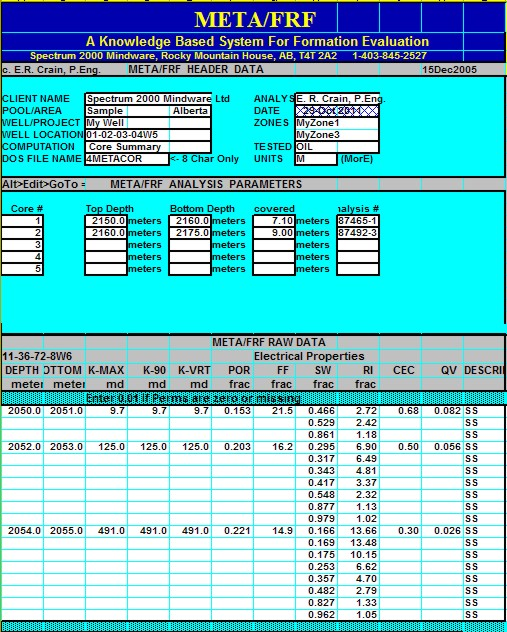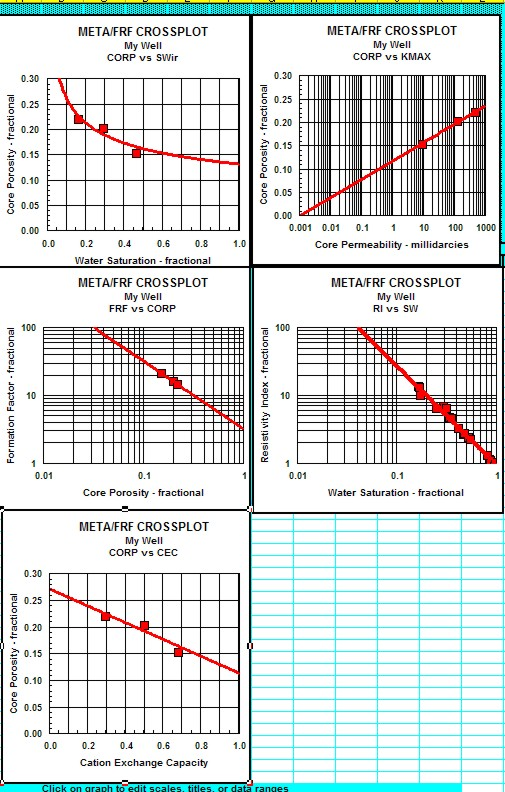Sample output from "META/FRF" spreadsheet for summarizing electrical properties.

Page Views ---- Since 01 Jan 2015
Copyright 2023 by Accessible Petrophysics Ltd.
CPH Logo, "CPH", "CPH Gold Member", "CPH Platinum Member", "Crain's Rules", "Meta/Log", "Computer-Ready-Math", "Petro/Fusion Scripts" are Trademarks of the Author